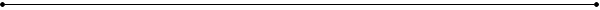// Counting from 10 to 50 in increments (steps) of 5: int x; for ( x=10; x <= 50; x = x+5 )                //**increment/decrement won't work here. {                                                              // x = x + 5 could also be written x += 5      cout<< "Loop counter value is " << x << ".\n"; }//Counting from 50 to 10 backwards in increments of 5: int x; for ( x=50; x >= 10; x = x-5 )                       // x = x - 5 could also be written x  -= 5 {      cout<< "Loop counter value is " << x << ".\n"; }// Declaring and assigning the startExpression within the for loop: //Counting down from 10 to Blast Off for ( int i = 10; i >= 1; i-- )               // notice the int within the parentheses {      cout<< i << '\n'; } cout<< "Blast off!\n";// Delcare loop body variables outside of the loop:  apstring child;  // if declared within the loop, these variables would be re-declared int age;             // ...each time the loop repeated, possibly causing problems. for (int ctr = 1; ctr <= 35; ctr++)            {      cout << "Enter the child's name: ";      cin >> child;      cout << "Enter the child's age: ";      cin >> age;      if ((age >= 6) && (age <=7))      {           cout <<"\n" <> num; cout << "Here are your stars:   \n"; for (int i = 1; i <= num; i++) {      cout << * << " "; }// Printing titles and for loops: // print titles OUTSIDE the loop to prevent multiple copies of the title //print even numbers from 1 to 20 with title cout << "Even numbers from 1 to 20: \n";           //title is outside loop for (int num = 2; num <= 20; num += 2)            //notice startExpression {      cout << num << " " ;                                     //prints the even numbers }